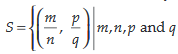# Consider the following relations:are integers such thatthen Option 1) is an equivalence relation but   is not an equivalence relation Option 2) neither nor is an equivalence relation Option 3) is an equivalence relation but  is not an equivalence relation Option 4) and both are equivalence relations

As we learnt in

Equivalence relation -

Any relation which is reflexive, symmetric and transitive is called an equivalence relation

-

given that qm = pn ,

Is is always true for (a, a) reflexive.

x = - 1

{0,  -1}

Correct option is 4.

Option 1)

is an equivalence relation but   is not an equivalence relation

This is an incorrect option.

Option 2)

neither nor is an equivalence relation

This is an incorrect option.

Option 3)

is an equivalence relation but  is not an equivalence relation

This is an incorrect option.

Option 4)

and both are equivalence relations

This is the correct option.

### Preparation Products

##### JEE Main Rank Booster 2021

This course will help student to be better prepared and study in the right direction for JEE Main..

₹ 13999/- ₹ 9999/-
##### Knockout JEE Main April 2021 (Subscription)

An exhaustive E-learning program for the complete preparation of JEE Main..

₹ 4999/-
##### Knockout JEE Main April 2021

An exhaustive E-learning program for the complete preparation of JEE Main..

₹ 22999/- ₹ 14999/-
##### Knockout JEE Main April 2022

An exhaustive E-learning program for the complete preparation of JEE Main..

₹ 34999/- ₹ 24999/-